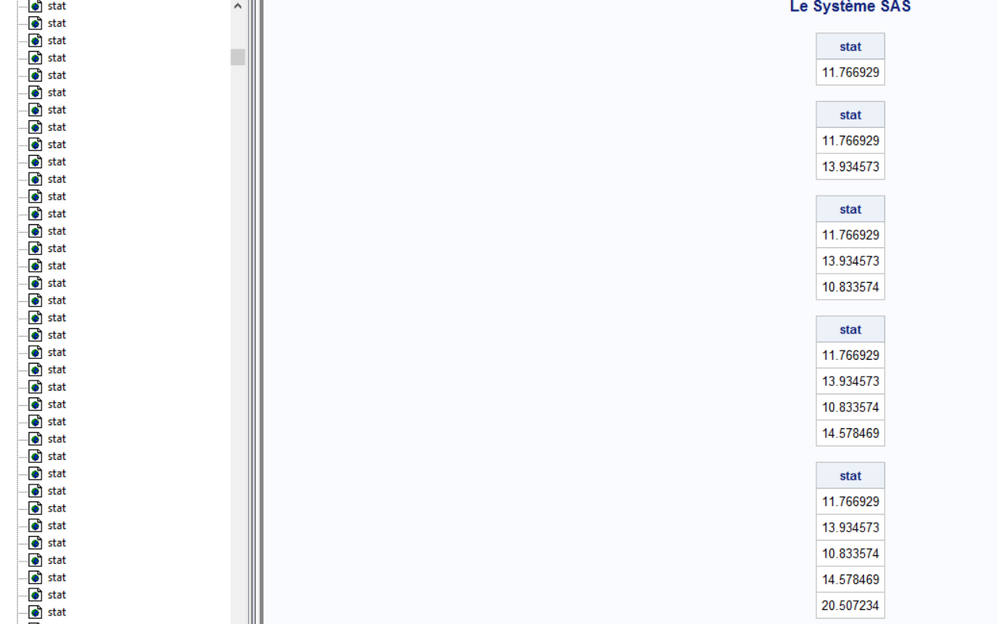## DO Loops : Struggling to save data in each iteration

Hello SAS Community,

Here are my functions :

```proc iml;

start White(X,Y);

DO i=1 to nrow(X);
X1_carré = X1_carré//X[i,1]*X[i,1];
end;

DO i=1 to nrow(X);
X2_carré = X2_carré//X[i,2]*X[i,2];
end;

DO i=1 to nrow(X);
X3_carré = X3_carré//X[i,3]*X[i,3];
end;

DO i=1 to nrow(X);
X4_carré = X4_carré//X[i,4]*X[i,4];
end;

DO i=1 to nrow(X);
X1X2_carré = X1X2_carré//X[i,1]*X[i,2];
end;

DO i=1 to nrow(X);
X1X3_carré = X1X3_carré//X[i,1]*X[i,3];
end;

DO i=1 to nrow(X);
X1X4_carré = X1X4_carré//X[i,1]*X[i,4];
end;

DO i=1 to nrow(X);
X2X3_carré = X2X3_carré//X[i,2]*X[i,3];
end;

DO i=1 to nrow(X);
X2X4_carré = X2X4_carré//X[i,2]*X[i,4];
end;

DO i=1 to nrow(X);
X3X4_carré = X3X4_carré//X[i,3]*X[i,4];
end;

XW = X||X1_carré||X2_carré||X3_carré||X4_carré||X1X2_carré||X1X3_carré||X1X4_carré||X2X3_carré||X2X4_carré||X3X4_carré;

n=nrow(X);
nw=nrow(XW);
X=J(n,1,1)||X;

XW=J(nw,1,1)||XW;
k=ncol(X);
kw=ncol(XW);

C=inv(X`*X);
beta_hat=C*X`*y;
resid=y-X*beta_hat;

resid_sq=resid#resid;

C_E=inv(XW`*XW);
b_hat_e=C_E*XW`*resid_sq;

Mean_Y=Sum(resid_sq)/nw;
SSR=b_hat_e`*XW`*resid_sq-nw*Mean_Y**2;
SSE=resid_sq`*resid_sq-b_hat_e`*XW`*resid_sq;
SST=SSR+SSE;
R_Square=SSR/SST;

White=nw*R_Square; /* NR² */
pvalue= 1 - probchi(White, kw-1);
*print(White);
*print(R_Square);
*print(W);
*print(pvalue);
return White;
finish White;

start ImanConoverTransform(Y, C);
X = Y;
N = nrow(X);
R = J(N, ncol(X));

do i = 1 to ncol(X);
h = quantile("Normal", rank(X[,i])/(N+1) );
R[,i] = h;
end;

Q = root(corr(R));
P = root(C);
S = solve(Q,P);
M = R*S;

do i = 1 to ncol(M);
rank = rank(M[,i]);
y = X[,i];
call sort(y);
X[,i] = y[rank];
end;
return( X );
finish;```

And this is the loop i want to work :

```		C = { 1.00  0.75 -0.70  0,
0.75  1.00 -0.95  0,
-0.70 -0.95  1.00 -0.2,
0     0    -0.2   1.0};

*do i = 1 to 10; /* Theoritically -------> Starting DO Loop here */
call randseed(2);
N = 500;
A = j(N,4); y = j(N,1);
distrib = {"Normal" "Lognormal" "Expo" "Uniform"};
do i = 1 to ncol(distrib);
call randgen(y, distrib[i]);
A[,i] = y;
end;

X = ImanConoverTransform(A, C);

call randseed(2);
B = j(4,1);
distrib = {"Normal"};
call randgen(B, distrib);

call randseed(2);
e = j(nrow(X),1);
distrib = {"Normal"};
call randgen(e, distrib);

/* nos résidus */

Y = X*B+e;

/* et donc notre Y */

FITWHITE = Y||X; /* pour la proc model */

stat = stat//White(X,Y);

print(stat);

*end;```

I'm going to explain my self briefly. The functions are basically, White test for Heteroskedasticity, and the Iman-Conover algorithm (proposed by Rick Wicklin). In themselves, they work perfectly and if don't try to make a DO Loop, it actually returns me the correct stats and pvalue.

The issue is when i try to make a loop. What i want is just for each iteration (1 to 10 for example) having the NR² (White=nw*R_Square;) for a random seed of number and stocking its value in a vector (stat = stat//White(X,Y); ).

So in this example, what i'm expecting by printing the stat variable is a vector of 10 rows with differents NR² values.

And... here is the result :That's weird and it seems to be repeated not then 10 times but actually 500 times !(maybe linked to the N under the first call randseed?)

I'll be glad to be helped on this one !

1 ACCEPTED SOLUTION

Accepted Solutions

## Re: DO Loops : Struggling to save data in each iteration

When you pass a matrix to a SAS/IML module, the matrix is passed by reference. This means that if you change the matrix inside the module, that change will affect the matrix that is passed in. IN the WHITE module, you have the statement

X=J(n,1,1)||X;

which adds a new column to X. Therefore, each time you call the WHITE function within a loop, the matrix gets wider.

Change that line to something like

Z = =J(n,1,1)||X;

and then use Z to estimate the parameters and to find the residuals.

2 REPLIES 2

## Re: DO Loops : Struggling to save data in each iteration

When you pass a matrix to a SAS/IML module, the matrix is passed by reference. This means that if you change the matrix inside the module, that change will affect the matrix that is passed in. IN the WHITE module, you have the statement

X=J(n,1,1)||X;

which adds a new column to X. Therefore, each time you call the WHITE function within a loop, the matrix gets wider.

Change that line to something like

Z = =J(n,1,1)||X;

and then use Z to estimate the parameters and to find the residuals.

## Re: DO Loops : Struggling to save data in each iteration

1. You can improve the efficiency of the WHITE function by using elementwise multiplication of vectors instead of DO loops when you form the quadratic interaction terms:

``````		X1_carré = X[,1] # X[,1];
X2_carré = X[,2] # X[,2];
X3_carré = X[,3] # X[,3];
X4_carré = X[,4] # X[,4];
X1X2_carré = X[,1] # X[,2];
X1X3_carré = X[,1] # X[,3];
X1X4_carré = X[,1] # X[,4];
X2X3_carré = X[,2] # X[,3];
X2X4_carré = X[,2] # X[,4];
X3X4_carré = X[,3] # X[,4];
XW = X||X1_carré||X2_carré||X3_carré||X4_carré||X1X2_carré||X1X3_carré||X1X4_carré||X2X3_carré||X2X4_carré||X3X4_carré;
``````

2. You can put the allocations and the call to RANDSEED outside of the loop:

``````call randseed(2);
N = 500;
A = j(N,4); y = j(N,1);
B = j(4,1);
e = j(nrow(X),1);
numSamples = 10;
stat = j(numSamples,1,.);  /* allocate result vector */
do i = 1 to 10; /* Theoritically -------> Starting DO Loop here */
...
stat[i] = White(X,Y);
end;
``````
From The DO Loop